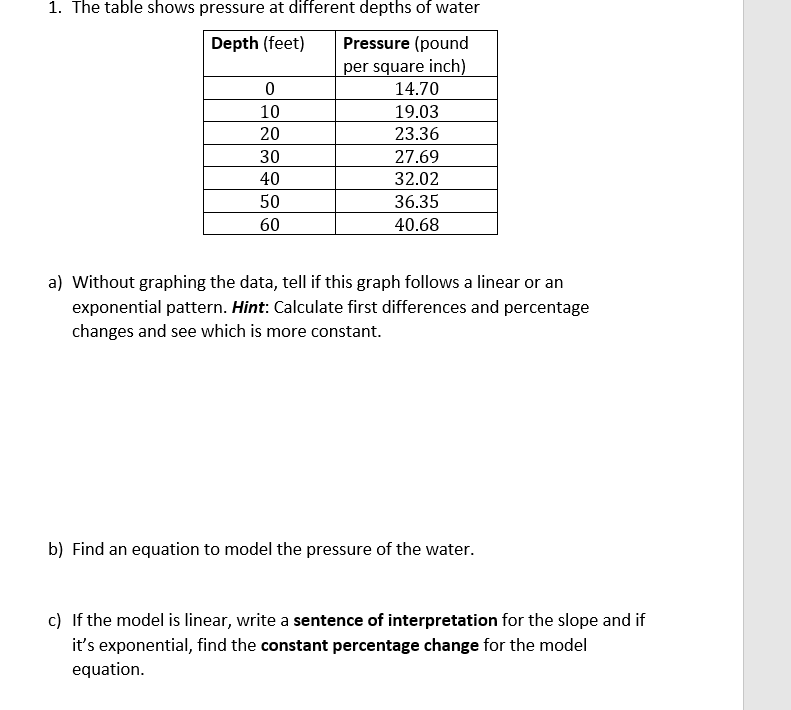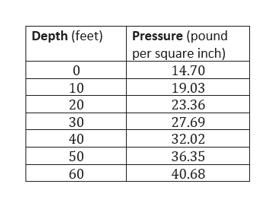# 1. The table shows pressure at different depths of waterPressure (poundDepth (feet)per square inch)14.70019.031023.36203027.694032.025036.356040.68a) Without graphing the data, tell if this graph follows a linear or anexponential pattern. Hint: Calculate first differences and percentagechanges and see which is more constant.b) Find an equation to model the pressure of the water.c) If the model is linear, write a sentence of interpretation for the slope and ifit's exponential, find the constant percentage change for the modelequation

Question
7 viewshelp_outlineImage Transcriptionclose1. The table shows pressure at different depths of water Pressure (pound Depth (feet) per square inch) 14.70 0 19.03 10 23.36 20 30 27.69 40 32.02 50 36.35 60 40.68 a) Without graphing the data, tell if this graph follows a linear or an exponential pattern. Hint: Calculate first differences and percentage changes and see which is more constant. b) Find an equation to model the pressure of the water. c) If the model is linear, write a sentence of interpretation for the slope and if it's exponential, find the constant percentage change for the model equation fullscreen
check_circle

Step 1

Given information:

The table shows pressure at different depths of water:help_outlineImage TranscriptioncloseDepth (feet) Pressure (pound per square inch) 14.70 0 10 19.03 23.36 20 30 27.69 40 32.02 50 36.35 60 40.68 fullscreen
Step 2

Calculation:

First difference is calculated by f(x+h)-f(x).

Step 3

Second Difference is calculated ...

### Want to see the full answer?

See Solution

#### Want to see this answer and more?

Solutions are written by subject experts who are available 24/7. Questions are typically answered within 1 hour.*

See Solution
*Response times may vary by subject and question.
Tagged in

### Functions